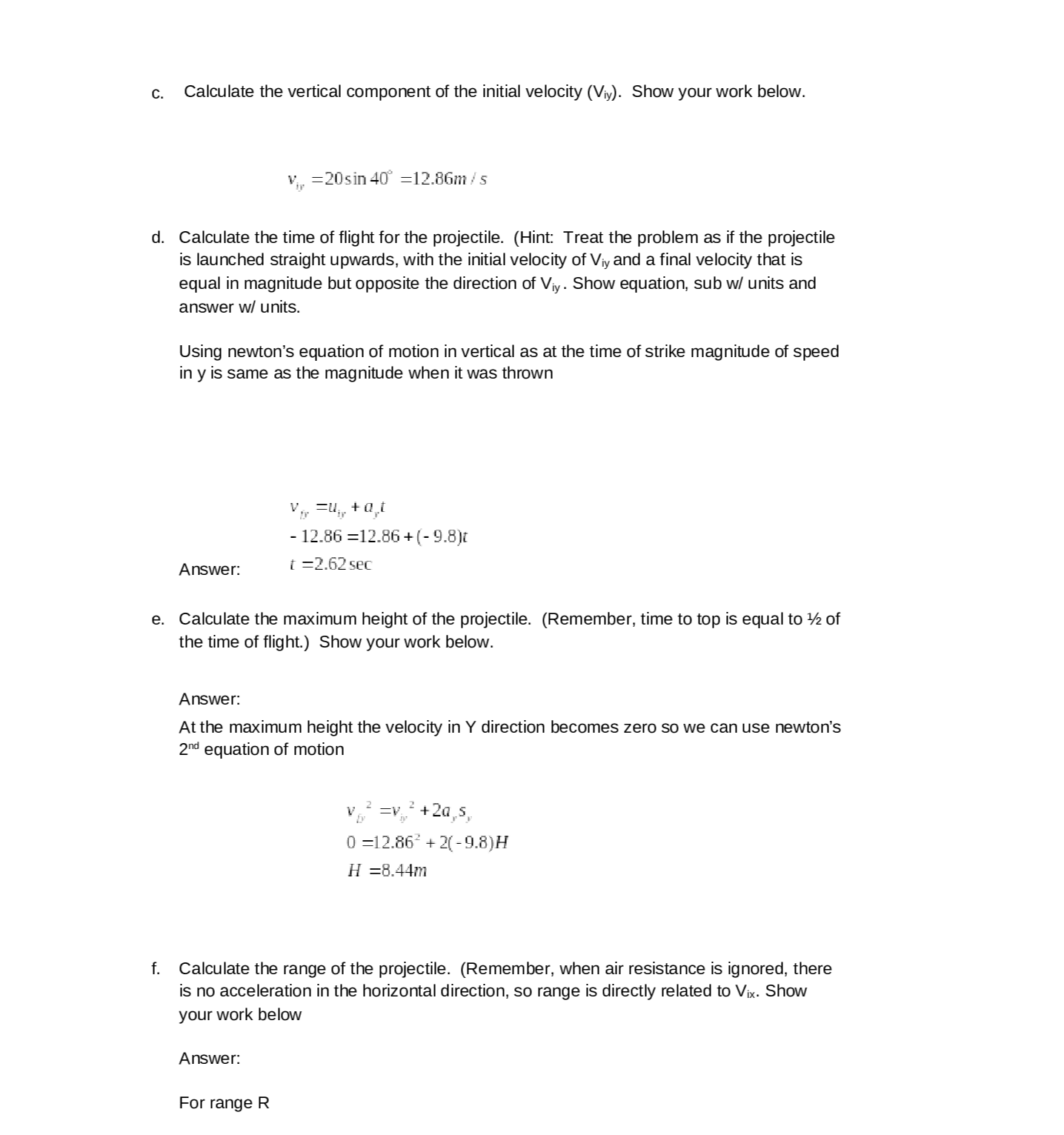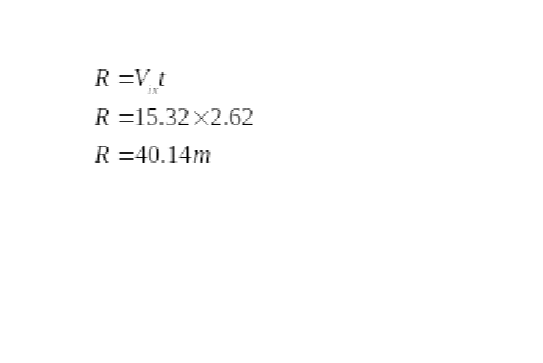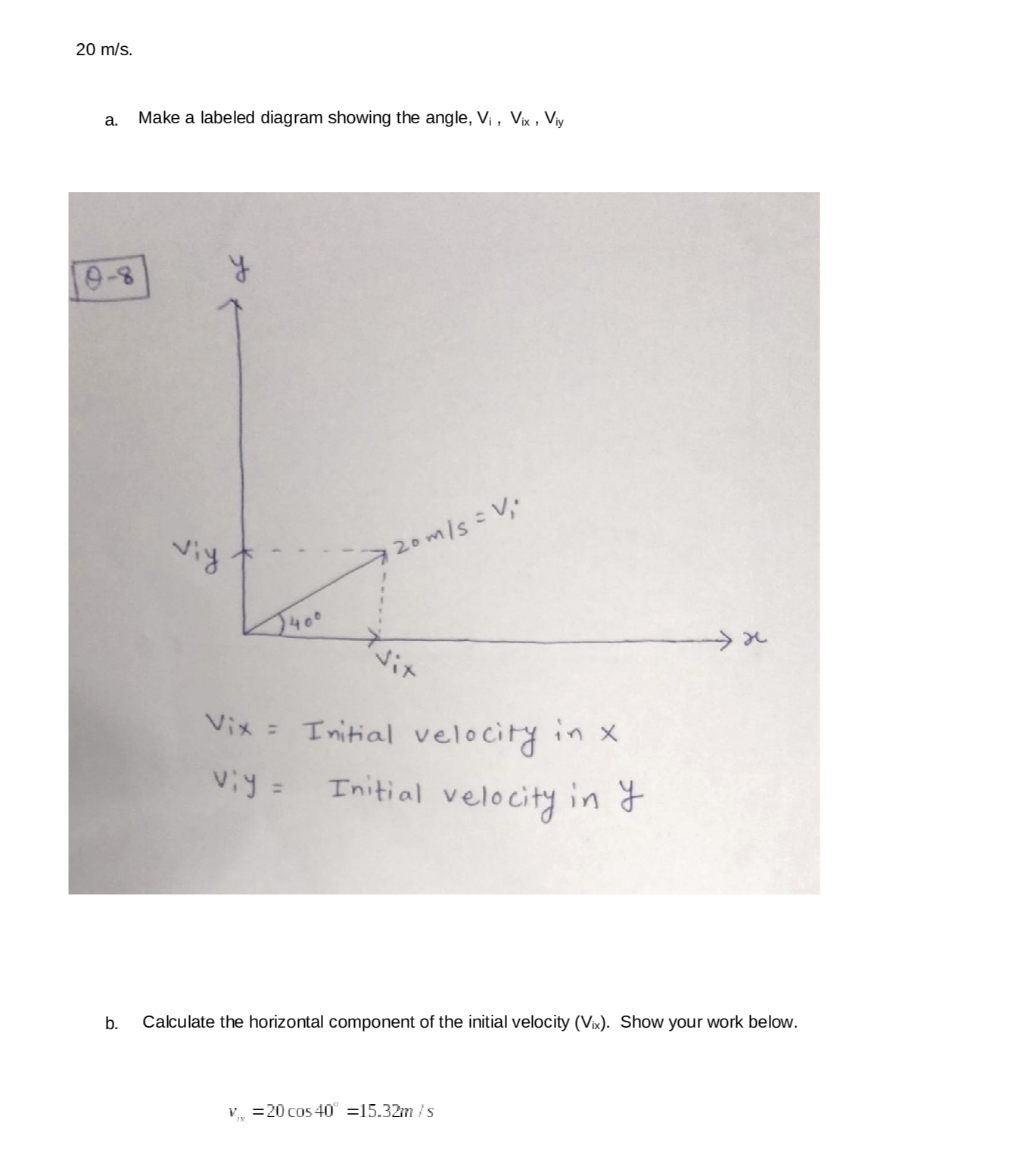Question

Mechanics

8. A projectile is fired at a 40° to the horizon, at a height of 0.0m, with an initial velocity of 20m/s.

а.Make a labeled diagram showing the angle, Vi , Vix , Viy

b.Calculate the horizontal component of the initial velocity (Vix). Show your work below.

С.Calculate the vertical component of the initial velocity (Viy). Show your work below.

d.Calculate the time of flight for the projectile. (Hint: Treat the problem as if the projectiles launched straight upwards, with the initial velocity of Viy and a final velocity that is equal in magnitude but opposite the direction of Viy. Show equation, sub w/ units and answer w/ units.

Calculate the maximum height of the projectile. (Remember, time to top is equal to ½ of the time of flight.) Show your work below.е.

f.Calculate the range of the projectile. (Remember, when air resistance is ignored, there's no acceleration in the horizontal direction, so range is directly related to Vix. Show your work belowVerified### Question 45701Mechanics

Q3 [45%] Consider the probability of finding a molecule in its ground state, P(e0),or first excited state, P(ɛ1), given the that energy separation between the two statesis 5 kJ/mol. You may assume that the states are non-degenerate (go = g1 = 1).
3] Calculate BAE for this system at 300 K.
[15%] Calculate the ratio of the excited and ground state populations P(ɛ1)/P(ɛo) at temperature T = 300 K
3. [15%] Show (by calculation) what would happen to the ratio if the temperature was increased by a factor of two.

### Question 45700Mechanics

Q2 [30%] Electron kinetic energies are often measured in units of electron-volts(1 eV = 1.6 x 10-19 J). When an aluminum plate is irradiated with UV light of 200nm wavelength, the ejected electrons are observed to have an average kinetic energy of about 2.11 eV. Use these results to
] Calculate the photon energy (in eV units).
Determine the electron binding energy O of aluminum (in eV units).

### Question 45699Mechanics

Q1 [25%] Consider a ball with mass m=5.0 kg on a hill with height h=10 m whichis inclined at an angle 0=30 degrees with respect to the horizontal axis and recallthat g=9.8 m/s².
] Calculate potential energy due to gravity11 1
Calculate the force on the ball due to gravity
Calculate the component of the force along the slope (z-axis).

### Question 45661Mechanics

What is the maximum mass of this water strider so that it can keep from breaking through the water surface? The strider has six legs

### Question 45660Mechanics

What is the maximum possible upward force on the foot due to surface tension of the water?

### Question 45659Mechanics

Blood plasma (at 37.0°C) is to be supplied to a patient at the rate of 2.80 × 10-6 m³/s. If the tube connecting the plasma to the patient's vein has a radius of 2.00 mm and a length of 60.8 cm, what's the pressure difference between the plasma and the patient's vein? Viscosity1.30 x 103 Pa·s.blood plasma is
39.55 Pa

### Question 45658Mechanics

Four identical sections of pipe are connected in various ways to pumps that supply water at the pressures indicated in the figure. The water exits at the right at 1.00 atm. Assume viscous flow. If the total volume flow rate in system Bis 0.0128 m³/s, what is the total volume flow rate in systemC?

### Question 45657Mechanics

Estimate the air speed above the wings. Density of air is 1.29 kg/m3.

### Question 45656Mechanics

An airplane flies on a level path. There is a pressure difference of 524 Pa between the lower and uppersurfaces of the wings. The area of each wing surface is about 100 m². The air moves below the wings ata speed of 80.5 m/s.
Estimate the weight of the plane.

### Question 45655Mechanics

The average speed of blood in the aorta is 0.320 m/s, and the radius of the aorta is 1.00 cm. There are about 2.00 × 10° capillaries with an average radius of 6.49 µm. What is the approximate average speed of the blood flow in the capillaries?

### Submit query

Getting answers to your urgent problems is simple. Submit your query in the given box and get answers Instantly.

### Submit a new QuerySuccess

Assignment is successfully created